Anúncio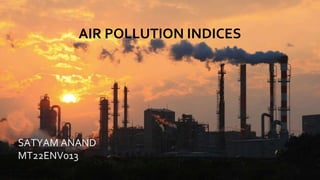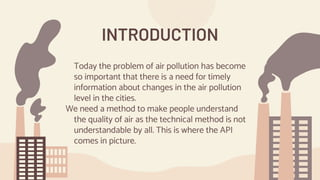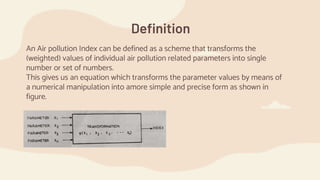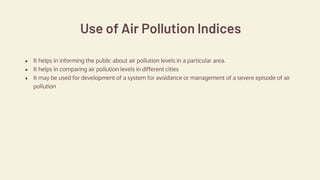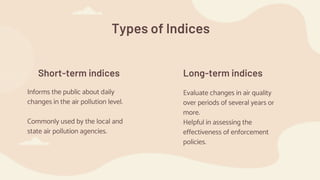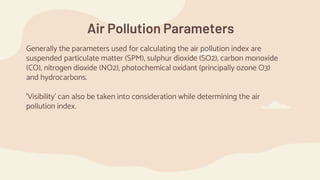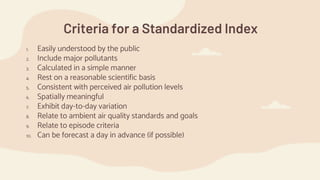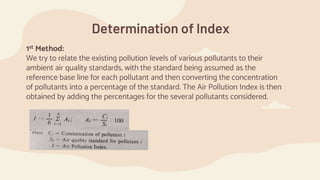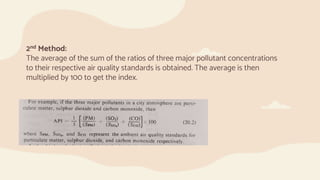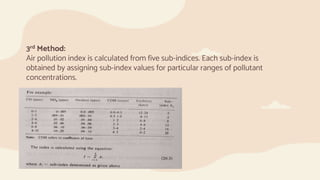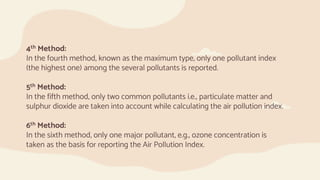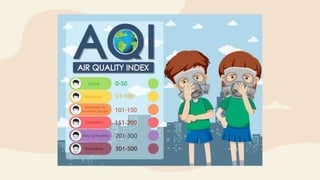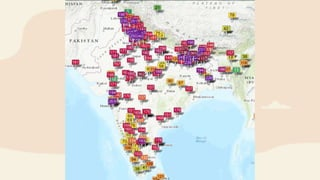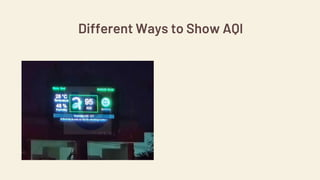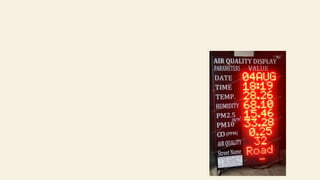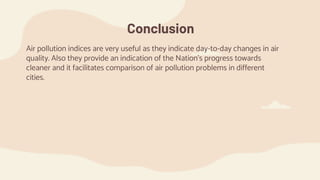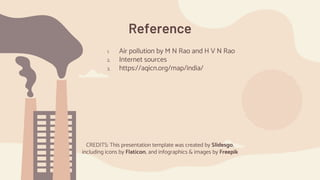1 de 18
Anúncio

### Air Pollution Indices.pptx

1. SATYAM ANAND MT22ENV013 AIR POLLUTION INDICES AIR POLLUTION INDICES SATYAM ANAND MT22ENV013
2. Today the problem of air pollution has become so important that there is a need for timely information about changes in the air pollution level in the cities. We need a method to make people understand the quality of air as the technical method is not understandable by all. This is where the API comes in picture. INTRODUCTION
3. Definition An Air pollution Index can be defined as a scheme that transforms the (weighted) values of individual air pollution related parameters into single number or set of numbers. This gives us an equation which transforms the parameter values by means of a numerical manipulation into amore simple and precise form as shown in figure.
4. Use of Air Pollution Indices ● It helps in informing the public about air pollution levels in a particular area. ● It helps in comparing air pollution levels in different cities ● It may be used for development of a system for avoidance or management of a severe episode of air pollution
5. Short-term indices Long-term indices Types of Indices Informs the public about daily changes in the air pollution level. Commonly used by the local and state air pollution agencies. Evaluate changes in air quality over periods of several years or more. Helpful in assessing the effectiveness of enforcement policies.
6. Air Pollution Parameters Generally the parameters used for calculating the air pollution index are suspended particulate matter (SPM), sulphur dioxide (SO2), carbon monoxide (CO), nitrogen dioxide (NO2), photochemical oxidant (principally ozone O3) and hydrocarbons. 'Visibility' can also be taken into consideration while determining the air pollution index.
7. Criteria for a Standardized Index 1. Easily understood by the public 2. Include major pollutants 3. Calculated in a simple manner 4. Rest on a reasonable scientific basis 5. Consistent with perceived air pollution levels 6. Spatially meaningful 7. Exhibit day-to-day variation 8. Relate to ambient air quality standards and goals 9. Relate to episode criteria 10. Can be forecast a day in advance (if possible)
8. Determination of Index 1st Method: We try to relate the existing pollution levels of various pollutants to their ambient air quality standards, with the standard being assumed as the reference base line for each pollutant and then converting the concentration of pollutants into a percentage of the standard. The Air Pollution Index is then obtained by adding the percentages for the several pollutants considered.
9. 2nd Method: The average of the sum of the ratios of three major pollutant concentrations to their respective air quality standards is obtained. The average is then multiplied by 100 to get the index.
10. 3rd Method: Air pollution index is calculated from five sub-indices. Each sub-index is obtained by assigning sub-index values for particular ranges of pollutant concentrations.
11. 4th Method: In the fourth method, known as the maximum type, only one pollutant index (the highest one) among the several pollutants is reported. 5th Method: In the fifth method, only two common pollutants i.e., particulate matter and sulphur dioxide are taken into account while calculating the air pollution index. 6th Method: In the sixth method, only one major pollutant, e.g., ozone concentration is taken as the basis for reporting the Air Pollution Index.
12. Rating Scale for Indices
13. Different Ways to Show AQI
14. Conclusion Air pollution indices are very useful as they indicate day-to-day changes in air quality. Also they provide an indication of the Nation's progress towards cleaner and it facilitates comparison of air pollution problems in different cities.
15. CREDITS: This presentation template was created by Slidesgo, including icons by Flaticon, and infographics & images by Freepik 1. Air pollution by M N Rao and H V N Rao 2. Internet sources 3. https://aqicn.org/map/india/ Reference
16. THANK YOU
Anúncio